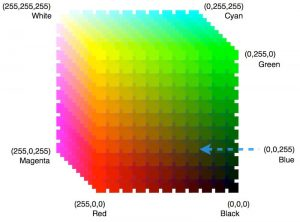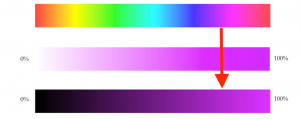# RGB vs. HSV

What can you see? On this page you will not find an optical illusion, but a comparison of two very important color models.In the RGB color model, color perceptions are reproduced by the addition of the three primary colors red, green and blue. It is used for self-luminous systems such as monitors.
The RGB color space is represented (however less clearly than in other color spaces) in the Cartesian coordinate system as a cube, in which the three colors are displayed on three axes with values of between 0-255.In the HSV color model, the colors are characterized by three values: the color value Hue, the color Saturation, and the brightness Value. It is often the preferred model because it resembles the human color perception.

What can you do? In the program you can play with both models and you can compare their corresponding values. Since in both color models all colors can be displayed, they can also be converted into each other.

• As long as rgb <-> hsv = 0, you can set the RGB values (for the left rectangle) and the HSV values (for the right rectangle) completely independently of each other.
• If you select rgb <-> hsv = 1, the freely selectable RGB values of the left-hand rectangle are converted to the corresponding HSV values and then used in the right-hand rectangle. The two rectangles should then be the same color.
• If you choose rgb <-> hsv = -1, the reverse is true: the freely selectable HSV values of the right-hand rectangle are converted to the corresponding RGB values and then used in the left-hand rectangle. The two rectangles should then again be the same color.